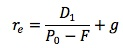### Seeing is believing!

Before you order, simply sign up for a free user account and in seconds you'll be experiencing the best in CFA exam preparation.

##### Subject 7. Flotation Costs
Flotation costs are the costs of issuing a new security, including the money investment bankers earn from the spread between their cost and the price offered to the public, and the accounting, legal, printing and other costs associated with the issue.

The amount of flotation costs is generally quite low for debt and preferred stock (often 1% or less of the face value), so we ignore them here. However, the flotation costs of issuing common stocks may be substantial, so they must be accounted for in the WACC. Generally, we calculate this by reducing the proceeds from the issue by the amount of the flotation costs and recalculating the cost of equity.Example 1

XYZ is contemplating issuing new equity. The current price of their stock is \$30 and the company expects to raise its current dividend of \$1.25 by 7% indefinitely. If the flotation cost is expected to be 9%, what would be the cost of this new source of capital?

Cost of external equity = (1.25 x 1.07) / (30 x (1 - 0.09)) + 0.07 = 11.9%

Without the flotation cost, the cost of new equity would be (1.25 x 1.07) / 30 + 0.07 = 11.46%.

Note that flotation costs will always be given, but they may be given as a dollar amount or as a percentage of the selling price.

This is a typical example found in most textbooks. One problem with this approach is that the flotation costs are a cash flow at the initiation of the project and affect the value of any project by reducing the initial cash flow. It is not appropriate to adjust the present value of the future cash flows by a fixed percentage. An alternative approach is to make the adjustment to the cash flows in the valuation computation.

Example 2

Continue with the above example. Assume that XYZ is going to raise \$10 million in new equity for a project. The initial investment is \$10 million and the project is expected to produce cash flows of \$4.5 million each year for 3 years.

Ignoring the flotation cost of issuing new equity, the NPV of the project will be -10 + 4.5/1.11461 + 4.5/1.11462 + 4.5/1.11463 = \$0.9093 million.

Now consider the flotation cost of 9%. The NPV, considering the flotation costs, is 0.9093 - 0.9 = \$0.0093 million.

However, if we use the "typical" approach, the NPV. considering the flotation costs, will be -10 + 4.5/1.1191 + 4.5/1.1192 + 4.5/1.1193 = \$0.8268 million.

Learning Outcome Statements

l. explain and demonstrate the correct treatment of flotation costs.

CFA® Level I Curriculum, 2020, Volume 4, Reading 33

User Comment
sheenalim In example 2, why when considering the flotation costs you subtract 0.9m from 0.9093m? Why 0.9m?
myron 0.9 million is 9% of 10 million. It's the flotation costs.
SDCFA Why cant 9% flotation cost be addedd to initial outflow.. its not a sunk cost and it will be spent initially ie it cant be deferred.. any comments?
sonus @SDCFA: you will still get the same result.
loisliu88 @sonus, I don't really get it. the result from the 2 examples are not the same.If including flotation cost in the discount rate, we get NPV of 0.8268. and if we use another approach by adjusting flotation cost to NPV, we get a NPV of 0.0093( 0.9093-0.9). can anyone explain?
ankursaxena -10 + -(10 * .09) + 4.5/(1.1146)^1 + ... = 0.0093
thekobe very important to have in mind that in every bond transactions flotation costs are front fees
johntan1979 So which exactly is the RIGHT approach? The one with the answer NPV = \$0.0093m or the "typical" approach with the answer NPV = \$0.8268m???

And for the record, Example 2 has nothing to do with bonds.
ankon yep
RamaG I would say, considering flotation costs as cost of equity is right approach, since the costs will need to be spread out over the period the equity capital benefits are realized ( till the point of equity retirement)
cgrimley I think we should calculate total flotation costs and include them as an initial cash outflow when calculating NPV. Comments?
birdperson i second johntan1979's Question
fobucina Like with many aspects in valuation, there usually isn't one way of doing things. To me, it makes more sense to treat the flotation costs as an initial cash outflow. In my perspective, the inherent operating risk of the company does not change with the occurrence of flotation costs. What do y'all think?
sshetty2 well they state that one PROBLEM associated with the calculation of flotation costs is that they ought not be calculated based solely on the initial cash flow but instead discounted based on the number of cash flows and the number years they are being discounted by suggesting that example two is more appropriate especially since the example they give illustrates how the inclusion of flotation costs will eat away nearly all of the NPV in example 1 instead of a reasonable amount in example 2
remo666 Can anyone help me with how did we arrive at 1.1146 please?! I just cant seem to understand.
MathLoser Those 2 examples are considered are Incorrect Treatments of Flotation costs in 2019.
The correct way to account for flotation costs is to adjust the initial project cost.
Calculate the dollar amount of the flotation cost attribute to the project and increase the initial cash outflow for the project by this amount.